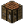Function (type)Tutorial: Functions An introduction to functions. Objective To gain an understanding of how Functions work, and how to use them Prerequisites A basic understanding of Variables.

What are functions?

Functions are a useful tool that helps simplify code, separating it by functionality and reducing repetitiveness. Understanding functions is key to becoming a successful coder. When a function is defined in Lua, it will most likely look like this:

function FunctionName()
--Do stuff
end

But may occasionally look more like a regular variable

Functionname=function()
--Do stuff
end

Both methods will yield the same result, so it's generally down to personal preference. Since functions are treated as normal variables, it is also possible to copy a function to a new variable

NewFunctionname=OldFunctionName

Note that here, we aren't using the "()" present in the other methods. This is because we're using OldFunctionName as a variable, and not calling it as a function.

How do I use functions?

More than likely, if you've followed the "Hello World" tutorial, you have already used functions. Without standard in-built functions such as write() and print(), it would be impossible to interact with a user. Whenever you see brackets after a variable name, you know it's a function.

For our first example, we will write a simple function that will use write()

function MyFunction(Str)
write(Str)
end
MyFunction("I am writing text to the screen!\n")

If you have successfully written and executed this script, it should write "I am writing text to the screen!" on the screen. Notice how the function has a variable name in the brackets. This is called an "argument", and will likely be crucial to your functions later. There can be any number of arguments, separated by commas, and arguments can be any type of variable, including other functions. It is also very important to call your function after you have defined it otherwise you will get an error like "attempt to call nil".

Add the following line to your code

MyFunction( true )

This will pass a boolean value to your function, and it will error as it reaches the write(), which can't use a boolean value. To fix this, we'll need to validate what type of variable is being given. Try adding another line so your code looks like this:

function MyFunction( Str )
if type( Str ) ~= "string" then return end
write( Str )
end
MyFunction( "I am writing text to the screen!\n" )
MyFunction( true )

The "type" functions will give you a string with the type of variable you give it. In this case, if the variable is not a string, it will run "return". Return will be described in more detail later in this tutorial, but for now we only need to know it ends the function, so any code after it will not be run. If you run your script now, you will see that there will be no error when it tries to use the boolean value. In fact, it will produce nothing at all, which may not be desirable. Edit the return line so it reads as follows

if type( Str ) ~= "string" then print("Bad argument #1 to MyFunction: String expected, got ".. type( Str ).."!\n") return end

If you run the code again, you will find it now gives an error when it reaches the boolean. The difference between this and the usual error() command called for a real error, is that this won't exit your code, and any line afterwards will still run as normal.

All this script really does is give a new name to the write() function, so there is a simpler way to do it. Before reading the following code, see if you can use your current knowledge to work it out for yourself.

The simplest way to get a new name for a function is as follows.

MyFunction = write

This means that any call to MyFunction() will act as write() did. Note that any changes to write() after this line will not edit MyFunction, which is why this method is often used to back up a built-in function before it's overwritten. As an example, someone may want to disable the write() function, but still have a way to use it when necessary.

MyFunction = write
write = function() MyFunction("This function has been disabled!\n") end

This will write "This function has been disabled!" every time someone tries to use write()

Return

Sometimes you will want a function to give a value back when it is done. This is the purpose of "return", it will return one or more values and end the function.

local function RandomNumber()
local rand = math.random(0,10)
return rand
end
local Num = RandomNumber()

This will set Num to a random number between 0 and 10. Notice how this time we are including the () for the function, this means we're calling the function rather than using it as a variable.